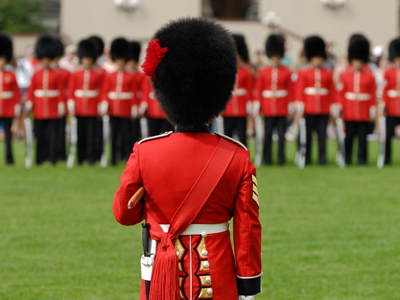Soldier on through this quiz for a question about soldiers!

# Fractions (Very Easy)

A fraction is a number that is not a whole number. It is written as one number divided another number, e.g. ¾. The top number, 3, is called the numerator and the bottom number, 4, is called the denominator: the denominator can NEVER be zero.

Fractions are read as follows: ⅜ is read as 'three eighths'. The numerator is read as an ordinary counting number, BUT the denominator is read as an ordinal number: third(s), fourth(s), fifth(s) and so on.

Fractions can have a strange effect on some people and they find using decimals preferable. Whatever your preference, it's definitely worth taking some time to play our 11-plus Maths quizzes about fractions. This will give you a basic understanding of these funny-looking numbers!

Have a go and do your best!

1.
In a battle, 110 of the general's army was destroyed. If he had 10,000 soldiers, how many soldiers were lost in the battle?
10,000
100
1,000
10
He lost 110 × 10,000 = 1,000 soldiers
2.
Peter's granny wanted to see if he understood his fractions. She asked him if he wanted 35 or 610 of the sweets. What did Peter say?
He said that he wanted 35 of the sweets
He said that it didn't matter what fraction of the sweets he took because the fractions were the same
He said that he wanted 610 of the sweets
He said that he wanted 35 of the sweets because 35 was closer to a half than 610
The fractions are the same. If you divide the denominator and the numerator in 610 by 2, you will get 35. The same is true if you multiply the denominator and the numerator in 35 by 2, you will get 610
3.
What does 38 + 28 equal?
516
58
316
316
If the denominators are the same, you can add the fractions by simply adding their numerators - it really is as easy as that!
4.
How many fifths (15) are there in 2?
10
5
20
25
There are five fifths in 1, so there are 5 × 2 = 10 fifths in 2. In general, the number in the denominator tells you how many fractions of that type are needed to make 1. For example, you need three thirds, 13, to make 1, but you need ten tenths, 110, to make 1 and so on. REMEMBER this stuff, and you won't get confused with fractions
5.
twenty-second thirty
twenty-two thirties
twenty-second thirtieth
twenty-two thirtieths
If you don't know your ordinal numbers, learn them now! For example, 20th = twentieth, 30th = thirtieth, 40th = fortieth and so on
6.
Which one of the fractions below is the biggest?
14
28
13
312
All the other fractions can be reduced to 14 which is smaller than 13 because you are dividing the same numerator, 1 in this case, by a smaller number: 3 in this case
7.
Which fraction lies between 26 and 46?
56
34
16
12
12 is the same as 36: multiply the numerator and denominator by 3
8.
What does 7949 equal?
1118
13
16
418
If the denominators are the same, you can subtract the fractions by simply subtracting their numerators - it really is as easy as that! You should get 39 BUT this can be reduced to 13 if you divide the denominator and the numerator by the SAME number, in this case 3
9.
Which one of the following fractions is the same as six-eighths (68)?
24
14
34
38
If you divide the denominator and the numerator by the SAME number, you can reduce the fraction to its simplest form. In this case, you divide the denominator AND the numerator by 2
10.
If you eat 57 of a cake, how much cake will be left?
77
27
37
127
There are seven sevenths in 1. If you have eaten 57, there can only be 27 left because 5 + 2 = 7: if the denominators are the same, you can add/subtract the fractions by simply adding/subtracting their numerators
Author:  Frank Evans